GFG App
Open AppBrowser
Continue

# Mapping Space Around Us – Visualizing Solid Shapes | Class 8 Maths

In Geometry, 3D shapes are known as three-dimensional shapes or solids, or solid shapes. 3D shapes or solid shapes have three different measures such as length, width, and height as its dimensions.

A polygon is a 2D shape with straight sides. A regular shape has all sides the same length and all interior angles the same size. An irregular shape has different length sides and/or interior angles. The only difference between 2D shapes and 3D shapes is that 2D shapes do not have a thickness or depth. Usually, 3D shapes are obtained from the rotation of the 2D shapes. The faces of the solid shapes are the 2D shapes. For example, the things we see in our usual life, like Brick, phones, tables, etc. are solid figures.

Various types of solid shapes are cubes, cuboids, prisms, pyramids, Platonic solids, torus, cone, cylinder, and sphere.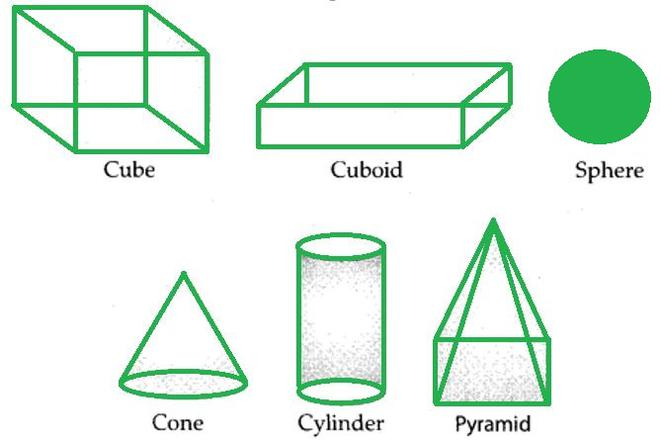Question: Write 5 examples of different solid shapes you see around yourself. Also, write their types.

Here are some examples of different solid shapes

(i) Duster – A Cuboid

(ii) Water Pipe – Cylinder

(iii) Football – Sphere

(iv) Rubik’s Cube – Cube

(v) Ice-Cream – Cone with a hemisphere

### Views of 3D Shapes using Nets

A net is a flattened out three-dimensional solid. It is the basic skeleton outline in two dimensions, which can be folded and glued together to obtain the 3D structure. Nets are used for making 3D shapes.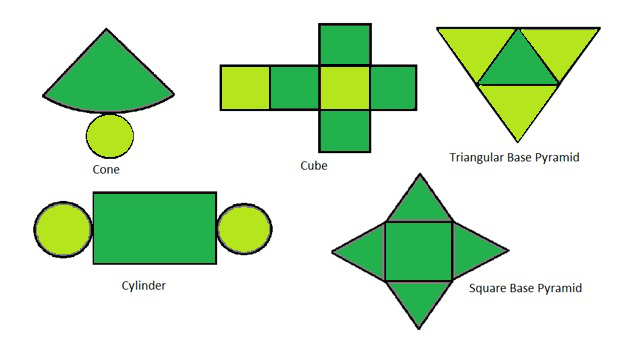Let us have a look at nets for different solids and their surface area and volume formula.

### Cuboid

A cuboid is also known as a rectangular prism. The faces of the cuboid are rectangular. All the angle measures are 90 degrees.

Example:

Take a matchbox. Cut along the edges and flatten out the box.

This is the net for the cuboid. Now if you fold it back and glue it together similarly as you opened it, you get the cuboid.

### Cube

A cube is defined as a three-dimensional square with 6 equal sides. All the faces of the cube have equal dimension

Example:

Take a cheese cube box and cut it out along the edges to make the net for a cube.

### Cone

A cone is a solid object that has a circular base and has a single vertex. It is a geometrical shape that tapers smoothly from the circular flat base to a point called the apex.

Example:

Take a birthday cap which is conical. When you cut a slit along its slant surface, you get a net for cone.

### Cylinder

A cylinder is a solid geometrical figure that has two parallel circular bases connected by a curved surface.

Example:

When you cut along the curved surface of any cylindrical jar, you get a net for the cylinder. The net consists of two circles for the base and the top and a rectangle for the curved surface.

### Pyramid

A pyramid, also known as a polyhedron. A pyramid can be any polygon, such as a square, triangle, and so on. It has three or more triangular faces that connect at a common point is called the apex.

Example:

The net for a pyramid with a square base consists of a square with triangles along its four edges.

### Views of 3D-Shapes

A 3D object can look different from different angles, so it can be drawn from different perspectives. For example, a brick can have the following views: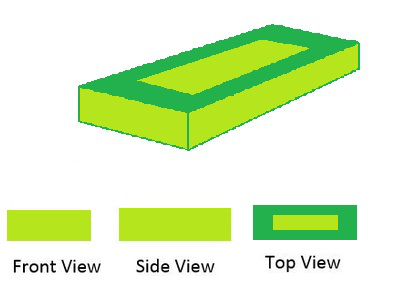### Mapping of Solid Shapes

You have been seeing maps since you were in primary classes. You have used the map to find different states, rivers, mountains, seas, and other places.

How do we read maps? What can we understand while reading a map? What information does a map have? Is it any different with a picture?

Now we will learn to read the maps. Look at the map given below: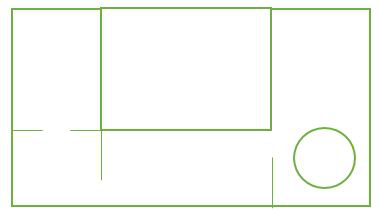What can we understand from the above map?

When we draw a picture, we try to represent reality with all details, whereas, a map only describes the position of an object, in relation to other objects. Now two different persons can give different thoughts about a picture, depending upon the position from which they are looking at the object.

But, this is not true in the case of a map. The map of the house remains the same for every position of the observer.

Now, look at the map in the following figure: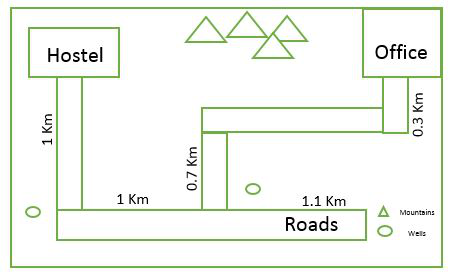From this map, can you tell-

(i) How far hostel is from his Office?

(ii) What landmarks do you see in the map?

This map is different from the earlier maps. Here, different symbols represent different landmarks. And longer line segments represent longer distances and shorter line segments represent shorter distances, i.e., a map has been drawn to a scale.

### Face

A face refers to any single flat surface of a solid object. Solid shapes can have more than one face. The polygonal regions which a solid is made of are called faces.

### Edges

An edge is a line segment on the boundary joining one vertex (corner point) to another. They serve as the junction of two faces. The faces meet at edges which are lines.

### Vertices

A point where two or more lines meet is called a vertex. It is a corner. The point of intersection of edges denotes the vertices. These edges meet at vertices which are points.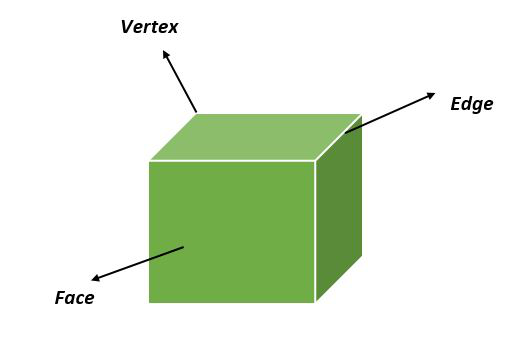Cube

Triangular Pyramid:

Side view of the pyramid will look like a triangular shape for the left and right sides.

Bottom of the pyramid has a triangle shape.

Faces = 4

Edges = 6

Vertices = 4

Square Pyramid: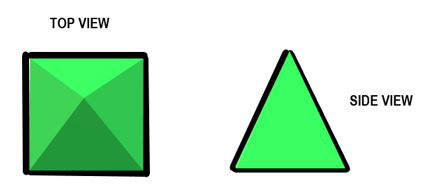Square Pyramid

Side view of pyramid will look like a triangular shape for left and right side.

Bottom of the pyramid has square shape.

Faces = 5

Edges = 8

Vertices = 5

### Polyhedrons

Polyhedron are straight-sided solids, which has the following properties:

• Polyhedrons should have straight edges.
• It should have flat sides are called the faces
• It must have the corners, called vertices

Like polygons in two-dimensional shapes, polyhedrons are also classified into regular and irregular polyhedrons and convex and concave polyhedrons.

The most common examples of polyhedral are cube, cuboid, pyramid, and prism. Some other examples of regular polyhedrons are tetrahedron, octahedron, dodecahedron, icosahedron, and so on.

These regular polyhedrons are also known as the platonic solids, whose faces are identical to each face.

For example, the most commonly used example of a polyhedron is a cube, which has 6 faces, 8 vertices, and 12 edges.

Such solids are called polyhedrons.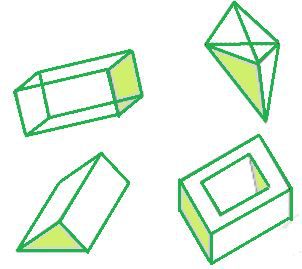### Curved solids or Non-Polyhedrons

Apart from polyhedrons, there are 3D shapes with curved shapes such as the sphere, cone, cylinder, etc. For example, cones have a circular base that narrows smoothly from the circular base to the point called the apex.

A cylinder is a three-dimensional shape consisting of two parallel circular bases, joined by a curved surface. A sphere is a geometrical object in three-dimensional space that is the surface of a ball. All these shapes have curved faces, and hence they are called curved solids or non-polyhedrons.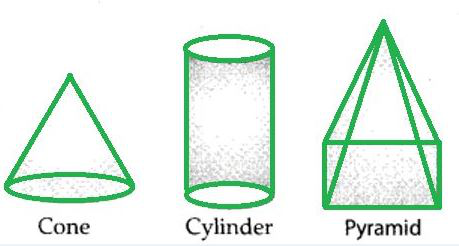### F + V – E = 2

Where

F = No. of Faces

V = No. of Vertices

E = No. of Edges

### Sample Problems on Euler’s Formula

Question 1. Using Euler’s formula find the unknown if faces are 20 and vertices are 12.

Solution:

Given

No. of Faces = F = 20

No. of Vertices =V =12

Find Out

No. of Edges = E =?

Using Euler’s formula

F + V – E = 2

Putting the value of F and V

20 + 12 – E = 2

32 – E = 2

E = 30

So No. of Edges are 30.

Question 2. Can a polyhedron have 18 edges, 7 faces, and 13 vertices?

Solution:

Given

No. of Faces = F = 7

No. of Vertices =V =13

No. of Edges = E =18

Using Euler’s formula

F + V – E = 2

Putting the value of F, V and E

13 + 7 – 18 = 2

2 = 2

L.H.S. is equals to R.H.S.

So a polyhedron can have 18 edges, 7 faces, and 13 vertices.

My Personal Notes arrow_drop_up